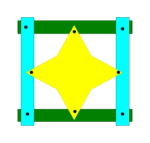# Algebraic Topology pamphlets?

I'm looking to self-learn some Algebraic Topology and have found the books I've looked at so far (ie. Hatcher) to be rather tome-like for my tastes. Does anyone know of a good slim lecture notes style book (or, indeed, an actual set of lecture notes) I can have a look at?

I need to catch up on the Cambridge part II course (I foolishly dropped it about half way through before realising the following term that I actually quite liked Geometry) for which the syllabus is as follows

The fundamental group

Homotopy of continuous functions and homotopy equivalence between topological spaces. The fundamental group of a space, homomorphisms induced by maps of spaces, change of base point, invariance under homotopy equivalence.

Covering spaces

Covering spaces and covering maps. Path-lifting and homotopy-lifting properties, and ther application to the calculation of fundamental groups. The fundamental group of the circle; topological proof of the fundamental theorem of algebra. Construction of the universal covering of a path-connected, locally simply connected space. The correspondence between connected coverings of X and conjugacy classes of subgroups of the fundamental group of X.

The Seifert–Van Kampen theorem

Free groups, generators and relations for groups, free products with amalgamation. Statement and proof of the Seifert–Van Kampen theorem. Applications to the calculation of fundamental groups.

Simplicial complexes

Finite simplicial complexes and subdivisions; the simplicial approximation theorem.

Homology

Simplicial homology, the homology groups of a simplex and its boundary. Functorial properties for simplicial maps. Proof of functoriality for continuous maps, and of homotopy invariance.

Homology calculations

The homology groups of Sn, applications including Brouwer’s fixed-point theorem. The Mayer-Vietoris theorem. Sketch of the classification of closed combinatorical surfaces; determination of their homology groups. Rational homology groups; the Euler–Poincar´e characteristic and the Lefschetz fixed-point theorem

http://tartarus.org/gareth/maths/notes/ has the notes from our year.

• This is great, thanks – tmh Jul 19 '12 at 17:57

My book Topology and Groupoids is one of few at this level to give an account of the van Kampen theorem with lots of applications (cell complexes, knots, Jordan Curve Theorem) and of covering spaces, both in terms of fundamental groupoids. (You also get orbit spaces, which you won't find elsewhere in English.) A pdf is available through the above web page.

A proof of a general version of the van Kampen theorem for the fundamental groupoid on a set of base points and a union of many open sets is available here (the proof is no harder than doing it for one base point!)

The pushout version for a union of two sets with two base points allows the computation of the fundamental group of the circle, which is, after all, THE basic example in algebraic topology. For that example, one base point is not enough, but two will do.shows a union of 3 spaces which requires $$8$$ base points to encapsulate the symmetry information.